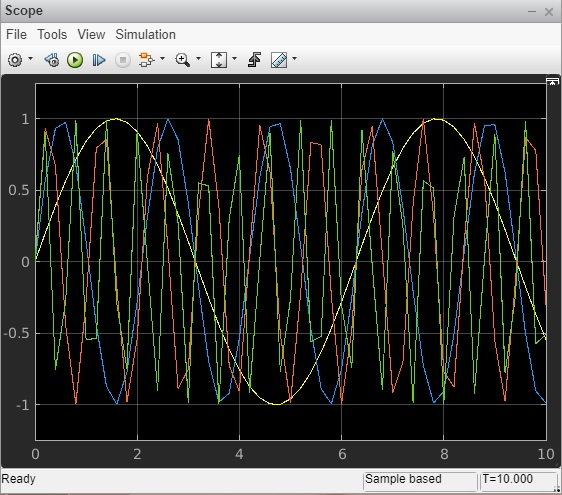# MATLAB Simulink - Build & Simulate Model

#### Data Preprocessing for Machine Learning using MATLAB

30 Lectures 4 hours

#### Image Processing Toolbox in MATLAB

17 Lectures 3 hours

#### Matlab - The Complete Course

Best Seller

37 Lectures 5 hours

We have seen the Simulink library browser and the blocks available in the library list. In this chapter, we are going to use the blocks to build a simple sine wave model.

Open Simulink and click on blank model as shown below −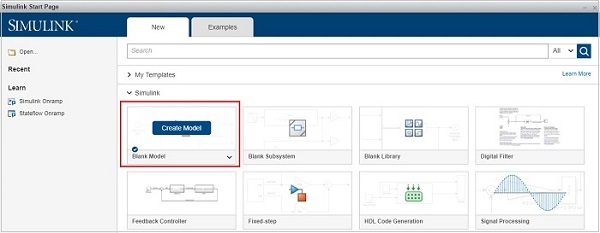The blank model will open a blank popup window as shown below −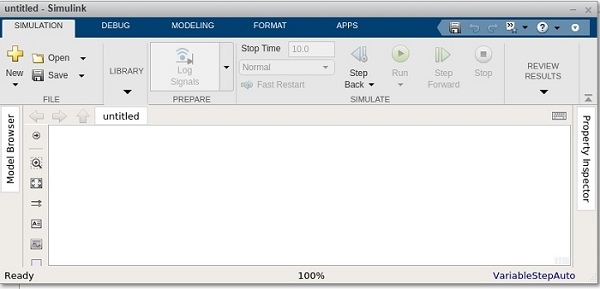Now, open the Simulink Library browser so that we can select the blocks.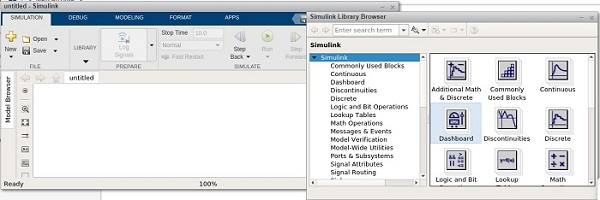The following screen will appear on your computer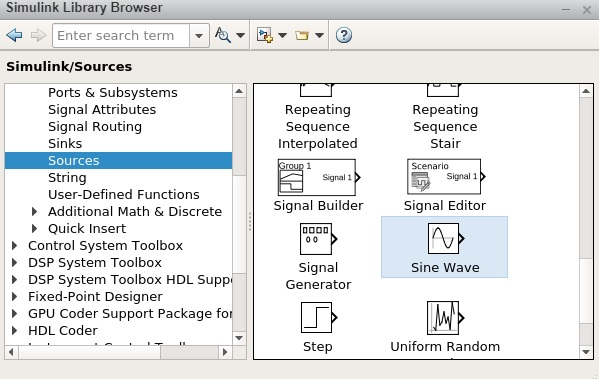To move the sine wave to your model, select the block and drag it inside your model workspace. We want to display the sine wave and here, we have taken four sign wave in our model workspace as shown below −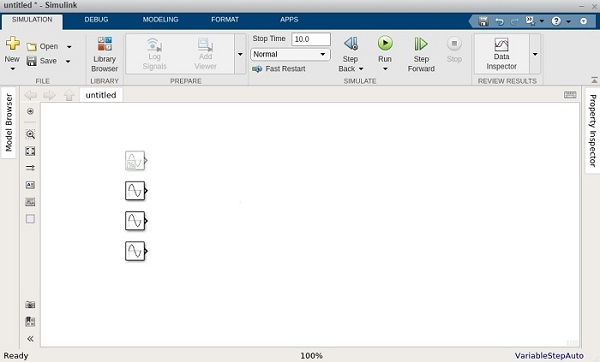Now we want to display the output of the signal, so let us use a Scope block from sinks library as shown below −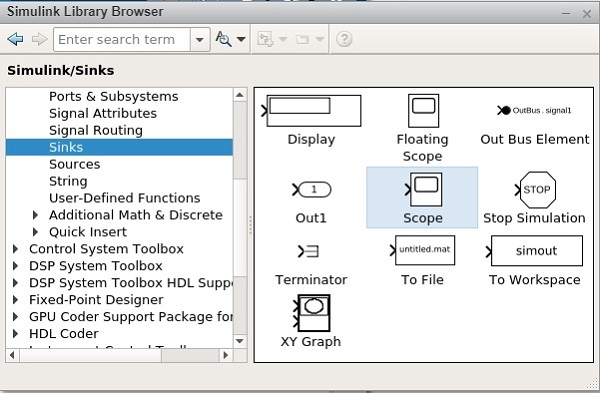Now select and drag the Scope block inside your model workspace.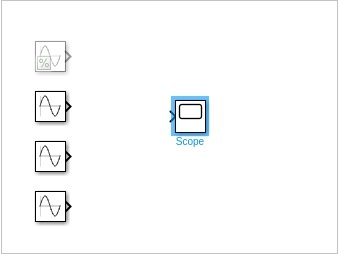The sine wave has one output and the scope block has one input. We have four sine waves displayed. We have to change the parameters of scope block to take four inputs.

Right click on scope block and click on Block Parameters and it will display the screen as shown below −Go to settings icon and change the input parameter from 1 to 4 as shown below −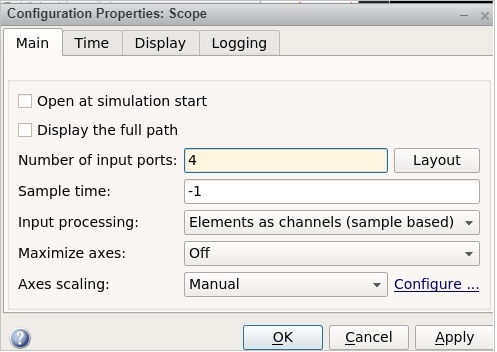Click on Apply to save the changes.

Let us now connect the sine wave to the scope block with arrows as shown below −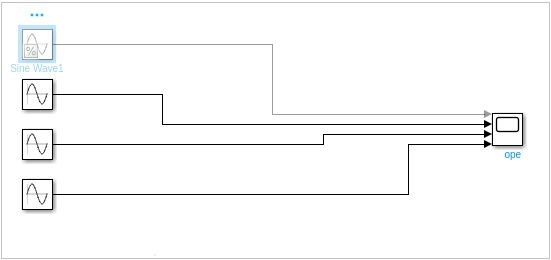We would like to change the frequency of each sine wave to a different one, so that we get a signal graph of different frequencies.

So right click on sine wave and open the Sine wave block parameters as shown below −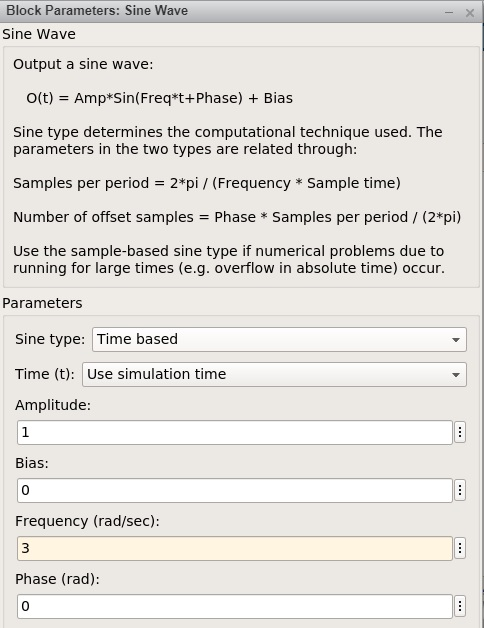We are going to keep the amplitude as 1 for all sine waves and the frequency of the first sine wave is 1, second one is 3, third one is 6 and the last one is 10.

Click on the Run button as shown below to see the sine wave.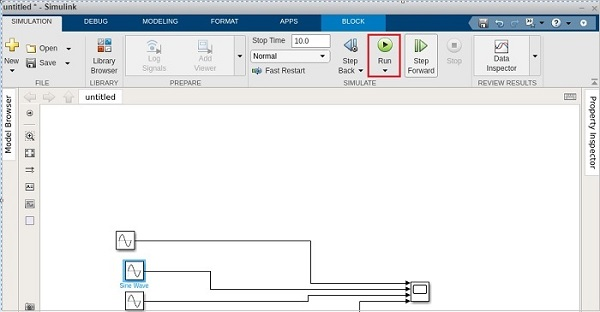Open the scope block parameters to see the sine wave as shown below.